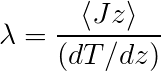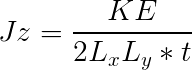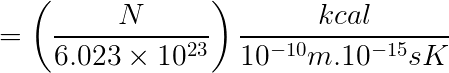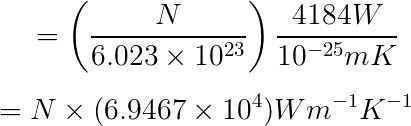# Thermal conductivity of a molecular system using RNEMD method (units?)

Dear all,

First of all apologies for a long mail.

I am trying to calculate thermal conductivity of a molecular melt using the RNEMD (Mu ̈ller-Plathe JCP 1997 & Zhang JPC 2005) method implemented in LAMMPS.
I work with real units and thus have the following conversion,-----------(1)-----------(2)

Real units

Energy: kcal/mol

(fix thermal/conductivity: This fix computes a global scalar which can be accessed by various output commands. The scalar is the cumulative kinetic energy transferred between the bottom and middle of the simulation box (in the edim direction) is stored as a scalar quantity by this fix. This quantity is zeroed when the fix is defined and accumulates thereafter, once every N steps. The units of the quantity are energy; see the units command for details. The scalar value calculated by this fix is “intensive”.)

Lx: Angstrom (10^-10 m)

time: fs (10-15 s)
Temperature: K
1 kcal/s = 4184 Watts
1mol = (N/N_A)N is the total number of molecules in the system and N_A the Avogadro’s number.

Here is the lammps input file,

1 Like

if you want to be sure of your parameters and conversion factors, you would simply run a simulation of a system where the result is known and try to reproduce that result.

axel.Dear Axel,

I have simulated water (benzene and ethanol), however with the conversion factor that I stated in my previous mail (probably not correct) there is no way I could get close to the reported values.

The units of each part of the thermal conductivity equation,Jz Numerator: (output of fix thermal/conductivity)

transferred kinetic energy --> kcal/mol --> kcal/(6.023e23)
(energy per molecule or atoms?)

``````Jz denominator:
area (Lx.Ly) --> Ang^2
time (step*dt) --> fs

(dT/dz) : (K/Ang)

Lambda = (kcal/mol)/(Ang. fs. K)

``````
• Conversion of (kcal/mol) / (Ang * fs * K) to W m-1 K-1

(1 kcal/s = 4184 Watts)

It would be great if someone experienced with calculating thermal conductivity of molecular system (real units) could guide me in this context.
I appreciate your time and help.

Thanks,
Tarak